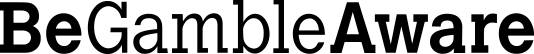# Odds and Probabilities

Whilst there are always exceptions, when a betting market is first formed the decimal odds that are available about any given competitor can be said to reflect the approximate probability of that competitor winning. The more likely it is that a given competitor will succeed, the lower the decimal odds for that competitor will be. Conversely, the less likely that the competitor will succeed, the higher the decimal odds for that competitor will be.

A bettor can use decimal odds to help him calculate the implied probability of any competitor winning an event by using the following formula:

100 / decimal odds = Implied Probability %

For example, if the home team in a football match is offered at decimal odds of 1.21, the implied probability of that team winning would be:

Home Win = 100 divided by 1.21 = 82.64%

If the Draw in this same match was offered at decimal odds of 21 and the Away Win was offered at decimal odds of 7, the implied probabilities would be:

Draw = 100 / 21 = 4.76%

Away Win = 100 / 7 = 14.28%

If the betting market was perfectly fair then the total of the implied probabilities for all possible outcomes would be a Book Total of 100%. However, that doesn’t usually happen. Instead, the Book Total of the implied probabilities usually exceeds 100%, and the excess represents the margin that gives the sportsbook its profit (often referred to as the over-round).

For example, when you add up the implied odds for the football match previously described, the Book Total is:

82.46% + 4.76% + 14.28% = 101.5%

In this scenario the over-round is 1.5%, which means that the bookmaker can expect to make 1.5% profit on this event as a whole. As far as over-rounds go, 1.5% is pretty good from the bettor’s point of view – some events give the bookie a profit margin of 10%, 20% and sometimes even more.

Of course, the 1.5% profit margin calculated here is just an average figure, and that’s because it is dependent on bets being placed according to the bookmaker’s expectations. If more bets than expected were placed on the Draw or Away Win result then the bookie might have to adjust the decimal odds offered about all possible outcomes so that he can ‘balance the book’ and protect his profits. Once such adjustments have started being made, using the decimal odds available as implied probabilities can quickly become misleading. For this reason it is essential that those who wish to calculate implied probabilities should always use the decimal odds on offer when the market was first formed, and not the decimal odds that become available when the forces of supply and demand have come into play.• Decimal Odds • Fractional Odds • Converting Odds • Probabilities • Ready Reckoner